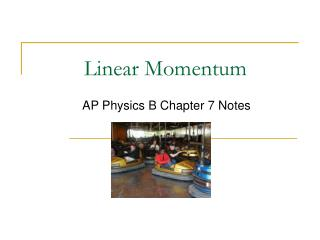# Linear Momentum - PowerPoint PPT PresentationDownload PresentationLinear Momentum

Linear Momentum
Download Presentation## Linear Momentum

- - - - - - - - - - - - - - - - - - - - - - - - - - - E N D - - - - - - - - - - - - - - - - - - - - - - - - - - -
##### Presentation Transcript

1. Linear Momentum AP Physics B Chapter 7 Notes

2. Linear Momentum • Which is easier to stop—a semi moving at 100 km/h or a VW beetle moving at 100km/h? • “Inertia in Motion”

3. Definition of Momentum • Momentum is a vector quantity and is defined as: • Units are kg∙m/s • The rate of change of momentum, ∆p is given by: • Another form on Newton’s second law, but now mass can change as well as velocity

4. Momentum Example • Example 7-2 pg. 169: Water leaves a hose at a rate of 1.5 kg/s with a speed of 20 m/s and is aimed at the side of a car which stops it. What is the force exerted by the water on the car? • If the water splashed back on from the car would the force be larger or smaller?

5. Conservation of Momentum • For isolated systems, total momentum is conserved, pbefore= pafter • An isolated system is one in which no outside forces act on the objects in the system

6. Conservation of Momentum Examples • P 6 pg.188: A 95 kg halfback moving at 4.1 m/s is tackled from behind by an 85 kg cornerback running at 5.5 m/s in the same direction. What is their speed immediately after the tackle? • Example7-4 pg. 172: Calculate the recoil velocity of a 5.0 kg rifle that shoots a 0.02 kg bullet at a speed of 620 m/s.

7. Collisions and Impulse • When objects collide, they are deformed due to large forces • Since , we can rearrange to: • This is the definition of impulse (∆p or J)—units are N∙s

8. Collisions and Impulse • Since the time of collision is so short, we can use average force • The area under the force-time graph is impulse—approximated by the square using F

9. Collisions and Impulse • Same impulse can result from: F∆t= m∆v F∆t= m∆v • Example 7-6 pg. 174:Find impulse for 70 kg man jumping from 3 m. Find force for 1 cm and 50 cm knees bending.

10. Collisions and Conservation of E and p • Two types of collisions—elastic and inelastic • Kinetic energy is conserved in elastic collisions only • Momentum is conserved in both types • Objects stick if completely inelastic

11. Elastic Collision Example • A handy formula to memorize for elastic 1-D collisions: • Example 7-7 pg. 176: Billiard ball A of mass m moving with speed v collides elastically with ball B, initially at rest and of equal m. What are the resulting speeds of the two balls? • P 26 pg. 189: A softball of mass 0.22 kg moving at 8.5 m/s collides head-on elastically with another ball initially at rest. The incoming ball bounces backwards with a speed of 3. m/s. Find the velocity and mass of the target ball.

12. Inelastic Collision Example • Only can use conservation of momentum for collision • Example 7-10 pg. 178: A projectile of mass m is fired at a block of mass M suspended by a pendulum. The projectile and pendulum together swing up to a maximum height h. Find the relationship between initial v of the projectile and h.

13. Two-D Collisions • AP B doesn’t cover, but C does and most college courses will (Section 7-7) • Must resolve into x- and y- components and then solve